# AP Physics C: Mechanics : Understanding Conservation of Energy

## Example Questions

### Example Question #9 : Work, Energy, And Power

A 0.8kg ball is dropped from rest from a cliff that is 150m high. Use conservation of energy to find the vertical velocity of the ball right before it hits the bottom of the cliff.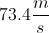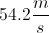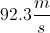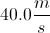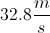Explanation:

The conservation of energy equation is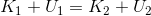.

The ball starts from rest so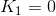. It starts at a height of 150m, so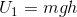.  When the ball reaches the bottom, height is zero and thus,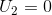and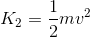.  The conservation of energy equation can be adjusted below.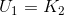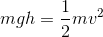Solve for v.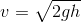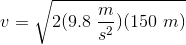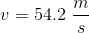### Example Question #1 : Work, Energy, And Power

Starting from rest, a skateboarder travels down a 25o incline that's 22m long. Using conservation of energy, calculate the skateboarder's speed when he reaches the bottom. Ignore friction.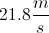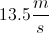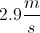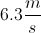Explanation:

Conservation of energy states that.

The skateboarder starts from rest; thus,and. At the bottom of the incline,and.Solve for v.Using trigonometry,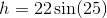.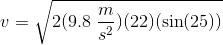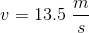### Example Question #1 : Understanding Conservation Of Energy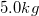bowling ball is dropped from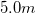above the ground. What will its velocity be when it is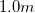above the ground?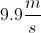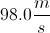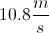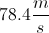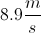Explanation:

Relevant equations: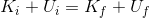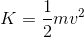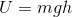Determine initial kinetic and potential energies when the ball is dropped.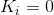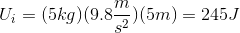Determine final kinetic and potential energies, when the ball has fallen toabove the ground.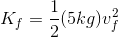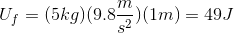Use conservation of energy to equate initial and final energy sums.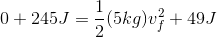Solve for the final velocity.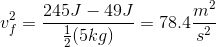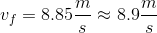### Example Question #2 : Understanding Conservation Of Energy

A solid metal object with mass of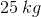is dropped from rest at the surface of a lake that is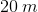deep. The water exerts a drag force on the object as it sinks. If the total work done by the drag force is -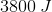, what is the speed of the object when it hits the sand at the bottom of the lake?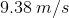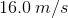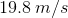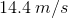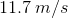Explanation:

This is a conservation of energy problem. First we have to find the work done by gravity. This can be found using: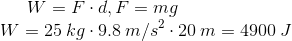It is given to us that the work done by the drag force is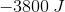which means that work is done in the opposite direction. We take the net work by adding the two works together we get,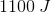of net work done on the block.

Since this is a conservation of energy problem, we set the net work equal to the kinetic energy equation: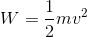is the mass of the block and we are trying to solve for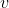.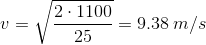### Example Question #131 : Mechanics Exam

If a roller coaster car is traveling at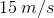when it is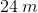above the ground, how fast is it going when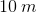above the ground?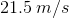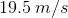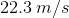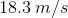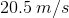Explanation:

This is a classic conservation of energy problem. We know that potential energy and kinetic energy both have to conserve. So we use the following equation: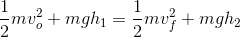What this equations says is, the sum of kinetic and potential energy is same at varying heights and velocities.

We can simplify this equation by cancelling out all the m terms.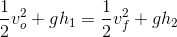We know all the terms except for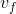, which is the final speed we are trying to solve for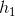, which is,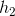isand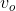is.

If we plug in all the numbers and solve for, we get.